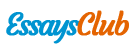# Assignment on Equations and Inequations

Autor:   •  September 16, 2017  •  Creative Writing  •  1,966 Words (8 Pages)  •  387 Views

Page 1 of 8

Note: Solve the assignment and send ONLY the exercises indicated by the teacher

1. Change the following propositions into one variable equations

1. Seven times a number equals five more than the number

2. Seventeen less four times a number equals twenty added to six times the number.

3. A number divided by four equals thirty added to nine times the number.

4. The addition of four pair and consecutive numbers is equal to 28

5. Five times a number less than three times the number equals the same number plus 45.

1. Solve the following equations

1. 2(x-3)² - 3(x+1)² + (x-5)(x-3) + 4(x²-5x+1) = 4x²-12

2. 5(x+2)/12 + 4/9 - (22-x)/36 = 3x - 20 - (8-x)/12 - (20 - 3x)/18

3. (x-2)/(x²+8x+7) = (2x-5)/(x²-49) - (x-2)/(x²-6x-7)

4. (x+a)/(x-a) - (x-a)/(x+a) = a(2x+ab)/(x²-a²)

5. √(2X-3) = 3X+5

6. (x-2)² - (2x+3)² = -80

7. x²/3 - (x-9)/6 = 3/2

8. |4x-3|> 2x - 5

1. Solve the following exercises from the Arya Book

1. Exercise 2-2. Numeral: 15, 31

2. Exercise 2-4. Numeral: 23

3. Exercise 3-3. Numeral: 17, 28

SOLUCION

Note: Solve the assignment and send ONLY the exercises indicated by the teacher

1. Change the following propositions into one variable equations

1. Seven times a number equals five more than the number

7x = 5 + x

1. Seventeen less four times a number equals twenty added to six times the number.

17-4x = 20+6x

1. A number divided by four equals thirty added to nine times the number.

X/4 = 30+9x

1. The addition of four pair and consecutive numbers is equal to 28

X+(x+2) +(x+4) +(x+6) =28

X es par.

1. Five times a number less than three times the number equals the same number plus 45.

5x-3x=x+45

2. Solve the following equations

1. 2(x-3)² - 3(x+1)² + (x-5)(x-3) + 4(x²-5x+1) = 4x²-12

2(x²-2(x) (3)+3²)-3(x²+2(x) (1)+1²)+(x-5) (x-3)+4x²-20x+4 = 4x²-12

2x²-12x+18-3x²-6x-3+x²-3x-5x+15+4x²-20x+4-4x²+12 = 0

2x²-3x²+x²+4x²-4x²-12x-6x-3x-5x-20x+18-3+15+4+12 = 0

-46x+46

X = -46/-46

X = 1

1. 5(x+2)/12 + 4/9 - (22-x)/36 = 3x - 20 - (8-x)/12 - (20 - 3x)/18

(5x+10)/12+4/9-(22-x)/36 = 3x-20-(8-x)/12-(20-3x)/18

(3(5x+10)+4(4)-22+x)/36 = (36(3x)-36(20)-3(8-x)-2(20-3x))/36

(15x+30+16-22+x)/36 = 108x-720-24+3x-40+6x/36

(16x+24)/36 = (117x-784)/36

36(16x+24) = 36(117x-784)

576x+864 = 4212x-28224

576x-4212x = -28224-864

-3636x = -29088

X = -29088/-3636

X = 8

1. (x-2)/(x²+8x+7) = (2x-5)/(x²-49) - (x-2)/(x²-6x-7)

(x-2)/(X+7) (x+1) = (2x-5)/(x+7) (x-7)-(x-2)/(x-7) (x+1)

(x-2)/(X+7) (x+1) = {(x+1) (2x-5)-(x+7) (x-2)}/(x-7) (x+1) (x+7)

(x-2)/(X+7) (x+1) = {(2x²-5x+2x-5)-(x²-2x+7x-14)}/(x-7) (x+1) (x+7)

(x-2)/(X+7) (x+1) = (2x²-5x+2x-5-x²+2x-7x+14)/(x-7) (x+1) (x+7)

(x-2)/(X+7) (x+1) = (x²-8x+9)/(x-7) (x+1) (x+7)

(x-2)/(X+7) (x+1) = (x-9) (x+1)/(x-7) (x+1) (x+7)

(x-2)/(X+7) (x+1) = (x-9)/(x-7) (x+7)

(x-2)/(x+1) = (x-9)/(x-7)

(x-2)(x-7) = (x-9) (x+1)

X²-7x-2x+14 = x²+x-9x-9

X²-7x-2x+14-x²-x+9x+9 = 0

-x+23 = 0

X= 23

1. (x+a)/(x-a)-(x-a)/(x+a) = a(2x+ab)/(x²-a²)

(x+a)/(x-a)-(x-a)/(x+a) = a (2x+ab)/(x-a) (x+a)

{(x+a) (x+a)-(x-a) (x-a)}/(x+a) (x-a) = a (2x+ab)/(x-a)(x+a)

(x-a)(x+a) {(x+a) (x+a)-(x-a) (x-a)}/(x+a) (x-a) = a (2x+ab)

...